Question

# The Let s1(t) and s2(t) be defined below:

The Let s1(t) and s2(t) be defined below: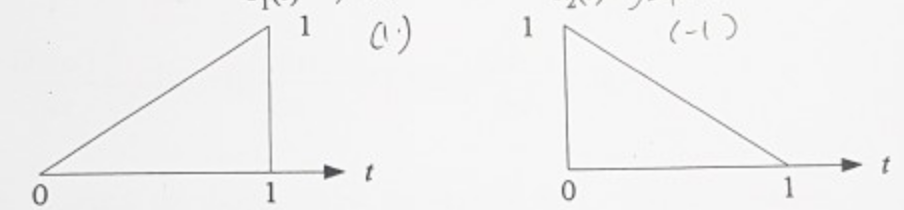(a) Find an orthonormal basis for S= span{s1(t) and s2(t)}.

(b) If y1(t) = 1, find and sketch ý1(t), the projection of y1(t) onto S.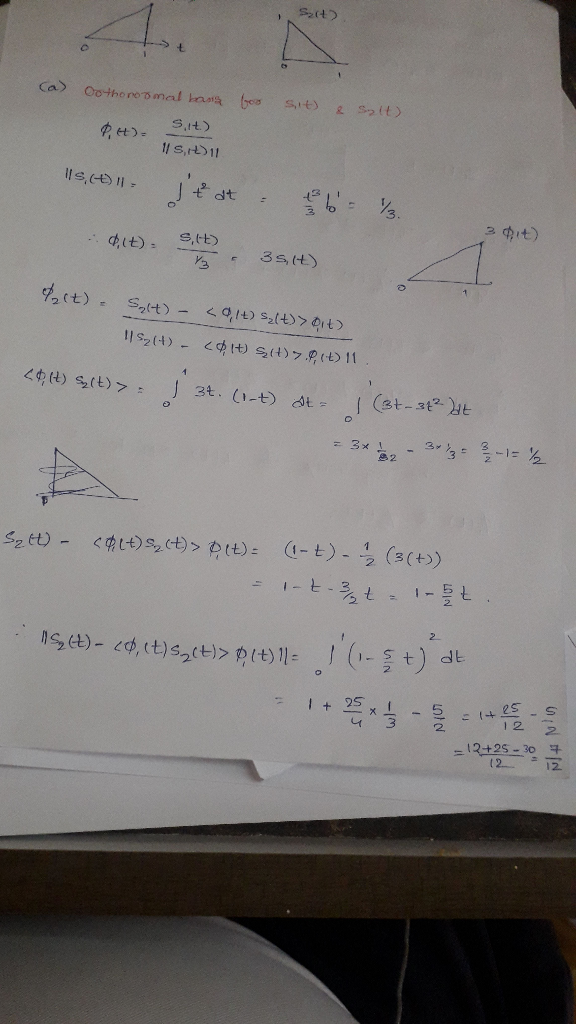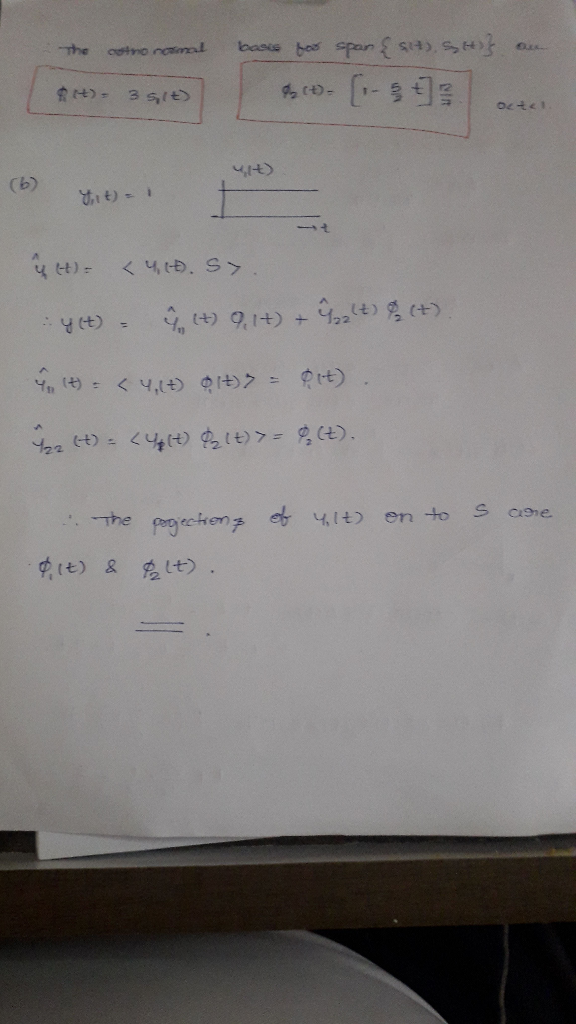#### Earn Coins

Coins can be redeemed for fabulous gifts.

Similar Homework Help Questions
• ### for the question, thanks for your help! 2. Let 2 -2 -11 1 3 S1 8...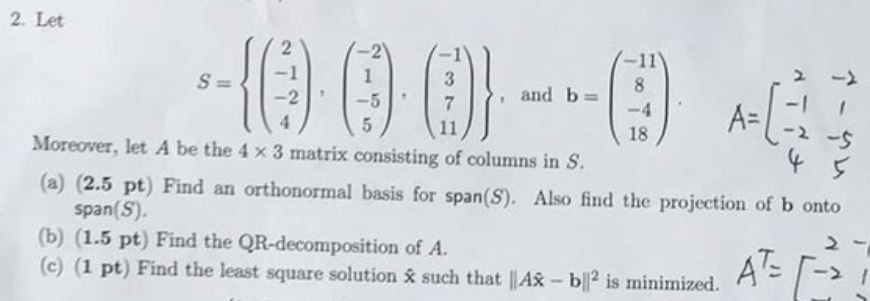for the question, thanks for your help! 2. Let 2 -2 -11 1 3 S1 8 and b -2 -5 7 A= -4 5 2-9 18 Moreover, let A be the 4 x 3 matrix consisting of columns in S (a) (2.5 pt) Find an orthonormal basis for span(S). Also find the projection of b onto span(S) (b) (1.5 pt) Find the QR-decomposition of A. (c) (1 pt) Find the least square solution & such that |A - bl2 is...

• ### Let V be a Hilbert space. Let S1 and S2 be two hyperplanes in V defined by Let be given. We con...

Let V be a Hilbert space. Let S1 and S2 be two hyperplanes in V defined by Let be given. We consider the projection of y onto , i.e., the solution of (1) (a) Prove that is a plane, i.e., if , then for any . (b) Prove that z is a solution of (1) if and only if and (2) (c) Find an explicit solution of (1). ( d) Prove the solution found in part (c) is unique. We...

• ### Consider the set of all polynomials of degree ≤3 in t defined over the interval [0,1]....

Consider the set of all polynomials of degree ≤3 in t defined over the interval [0,1]. (a) Find an orthonormal basis for this space. (b) Find the projection of the polynomial t^4 onto this space.

• ### 2. Consider R with the weighted inner product = [wn, u, tva, teal"). [ruh, t', talT and w Find the orthogonal projection of w = [1, 2,-1,2]T onto the span of ui-|1,-1, 2, 5]T and u2 [2,1,...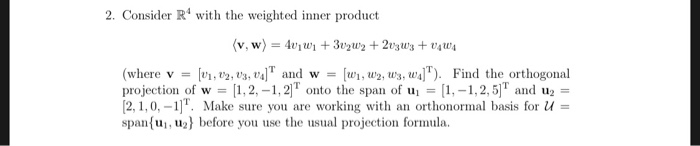2. Consider R with the weighted inner product = [wn, u, tva, teal"). [ruh, t', talT and w Find the orthogonal projection of w = [1, 2,-1,2]T onto the span of ui-|1,-1, 2, 5]T and u2 [2,1,0,-]. Make sure you are working with an orthonormal basis for u span(u, u2 before you use the usual projection formula. 2. Consider R with the weighted inner product = [wn, u, tva, teal"). [ruh, t', talT and w Find the orthogonal projection of...

• ### Let Ps have the inner product given by evaluation at -2, -1, 1, and 2. Let po(t)-1. P,()-t, and p...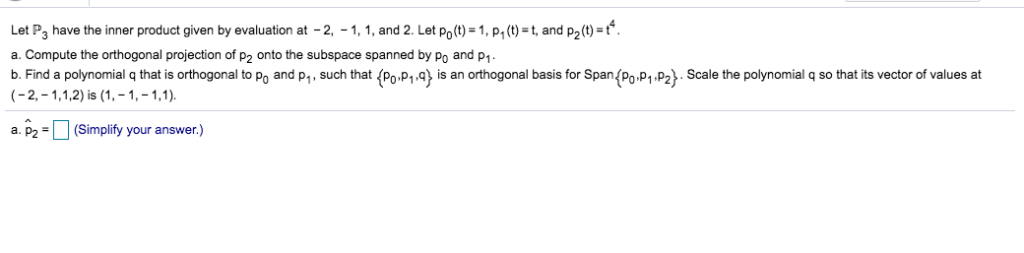Let Ps have the inner product given by evaluation at -2, -1, 1, and 2. Let po(t)-1. P,()-t, and p20)- a. Compute the orthogonal projection of p2 onto the subspace spanned by Po and P1 b. Find a polynomial q that is orthogonal to Po and p,, such that Po P is an orthogonal basis for Span(Po P1, P2). Scale the polynomial q so that its vector of values at a2(Simplify your answer.) Let Ps have the inner product given...

• ### 1. Let us consider a digital binary communication system, in which the fol- lowing signal s1(t)...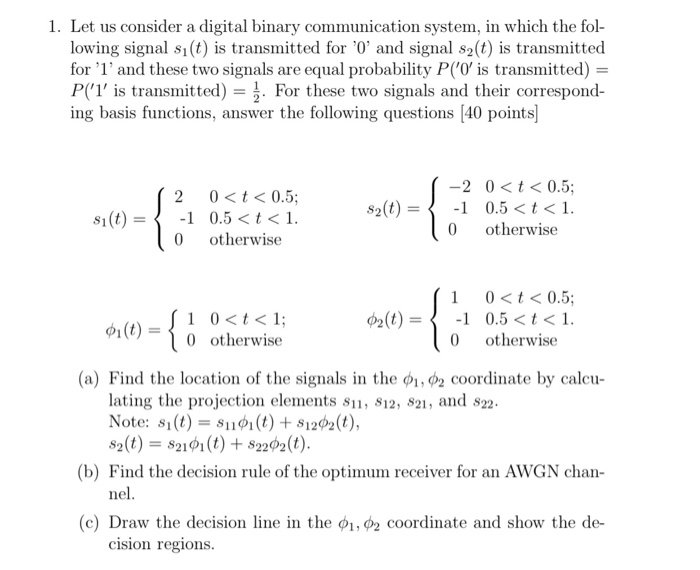1. Let us consider a digital binary communication system, in which the fol- lowing signal s1(t) is transmitted for '0' and signal s2(t) is transmitted for '1' and these two signals are equal probability P('O' is transmitted) P(1' is transmitted). For these two signals and their correspond- ing basis functions, answer the following questions [40 points -2 0t<0.5; 0<t<0.5 -1 0.5 <t< 1 2 s2(t) -1 0.5<t1. s1(t) otherwise 0 otherwise 0 0<t<0.5; -1 0.5 <t1. otherwise 1 10t<1 0...

• ### 6. Let P be the subspace in R 3 defined by the plane x − 2y...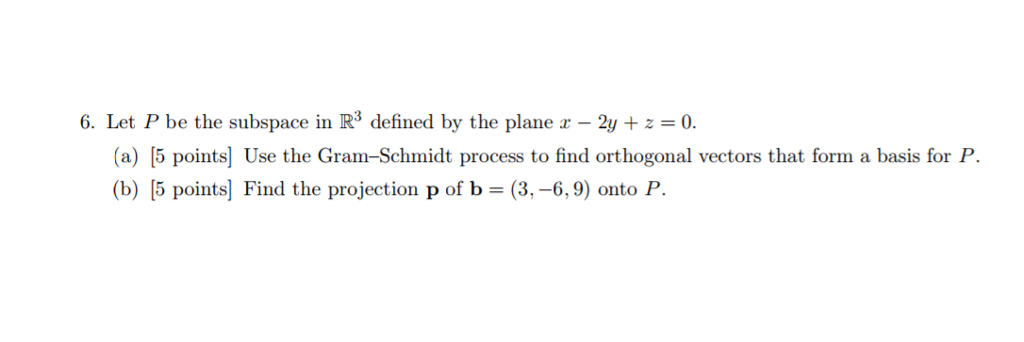6. Let P be the subspace in R 3 defined by the plane x − 2y + z = 0. (a) [5 points] Use the Gram–Schmidt process to find orthogonal vectors that form a basis for P. (b) [5 points] Find the projection p of b = (3, −6, 9) onto P. 6. Let P be the subspace in R3 defined by the plan 2y+z0 (a) [5 points] Use the Gram-Schmidt process to find orthogonal vectors that form a basis...

• ### 0. For n E N, n > 1, let s, be defined by 8. *Let s1...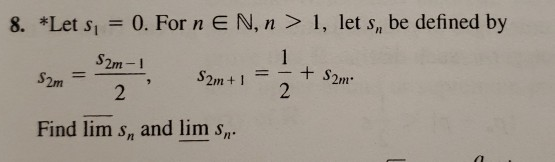0. For n E N, n > 1, let s, be defined by 8. *Let s1 1 +S2m 2 S2m-1 S2m+1 \$2m 2 Find lim s, and lim s,.

• ### 12) Let S1 be a countable set, S2 a set that is not countable, and S1...

12) Let S1 be a countable set, S2 a set that is not countable, and S1 ⊂ S2. a) Show that S2 must then contain an infinite number of elements that are not in S1. b) Show that in fact S2 − S1cannot be countable.

• ### Let T: P1 → P2 be a linear transformation defined by T(a + bx) = 3a...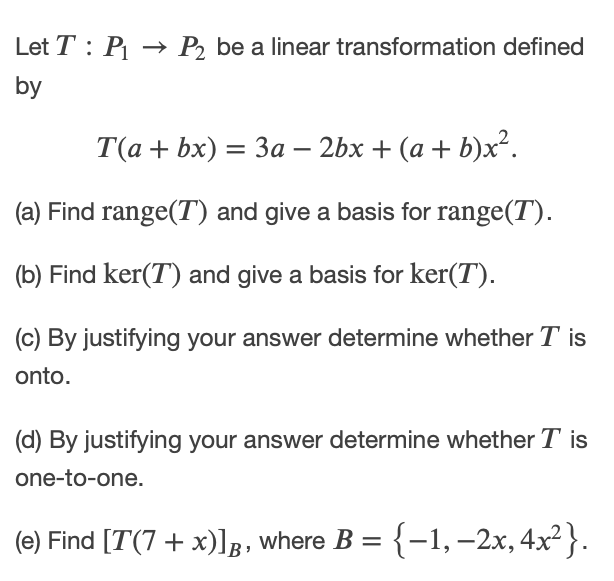Let T: P1 → P2 be a linear transformation defined by T(a + bx) = 3a – 2bx + (a + b)x². (a) Find range(T) and give a basis for range(T). (b) Find ker(T) and give a basis for ker(T). (c) By justifying your answer determine whether T is onto. (d) By justifying your answer determine whether T is one-to-one. (e) Find [T(7 + x)]], where B = {-1, -2x, 4x2}.